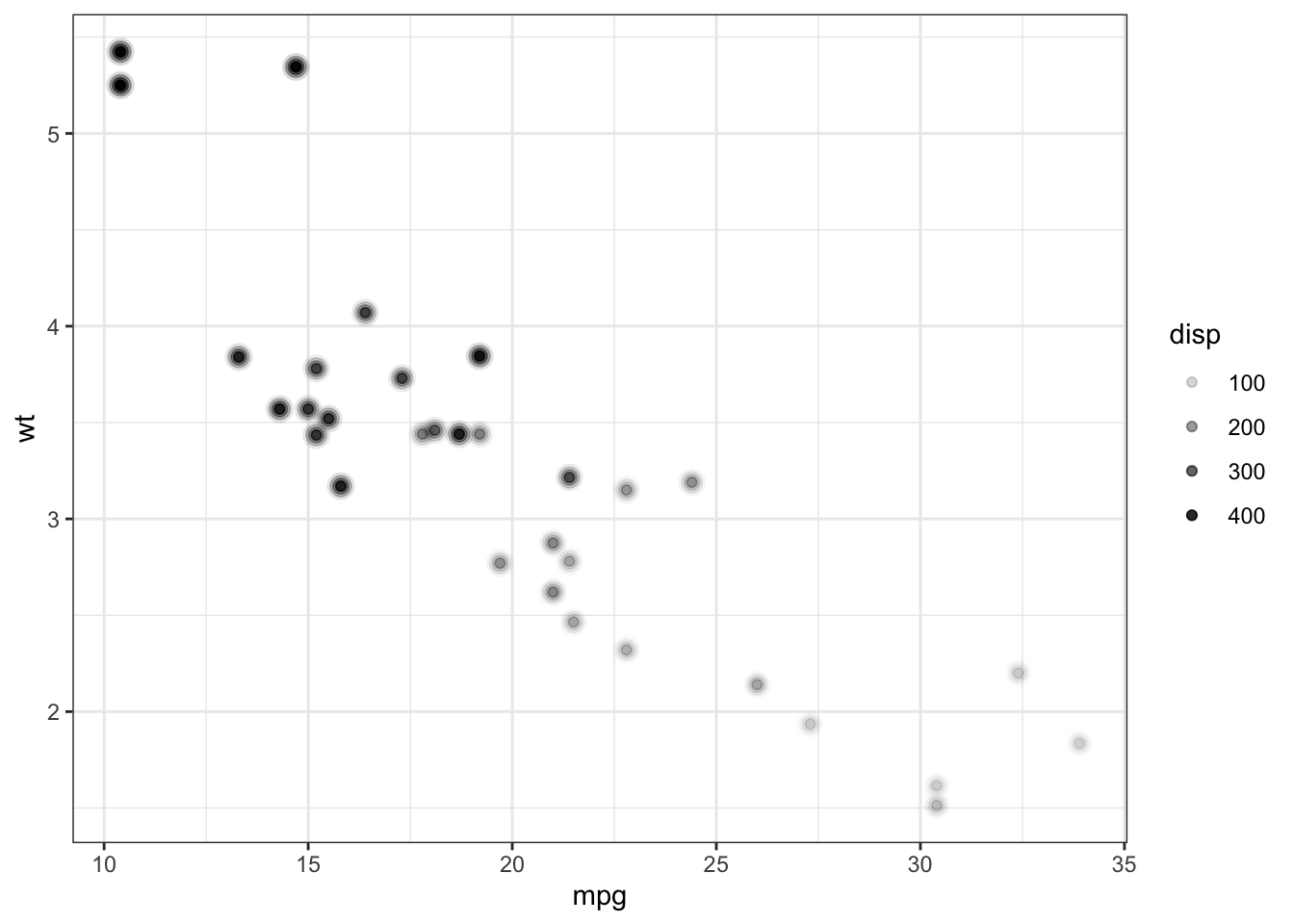# geom_blurry() - proof of concept

Emil Hvitfeldt wondered on twitter whether there was a convenient way to blur points in ggplot.

I created a proof-of-concept geom to plot blurry points. Not-at-all configurable at the moment, but it shows that it could be an interesting way of showing … something. Maybe uncertainty?

ggplot(mtcars) +
geom_blurry(aes(mpg, wt, alpha = disp)) +
theme_bw()## Ideas

• Make blur an aesthetic e.g. aes(x = wt, y = mpg, blur = disp)
• Set a blur size limit? i.e. some way to set the extra radius for the blur e.g. geom_blurry(aes(...), blur_size = 4)
• Would this be possible to do as a stat_blurry() so that it could work with multiple geoms. Somehow. No idea at the moment.
• This is probably do-able directly in grid somehow?

## Appendix: Code

The code used is included below and available as a gist on github

geom_blurry <- function(mapping = NULL, data = NULL,
stat = "identity", position = "identity",
...,
na.rm = FALSE,
show.legend = NA,
inherit.aes = TRUE) {
layer(
data = data,
mapping = mapping,
stat = stat,
geom = GeomBlurry,
position = position,
show.legend = show.legend,
inherit.aes = inherit.aes,
params = list(
na.rm = na.rm,
...
)
)
}

GeomBlurry <- ggproto(
"GeomBlurry", Geom,
required_aes = c("x", "y"),
non_missing_aes = c("size", "shape", "colour"),
default_aes = aes(
shape = 19, colour = "black", size = 1.5, fill = NA,
alpha = NA, stroke = 0.5
),

draw_panel = function(data, panel_params, coord, na.rm = FALSE) {
if (is.character(data$shape)) { data$shape <- translate_shape_string(data$shape) } coords <- coord$transform(data, panel_params)

my_alpha <- coords$alpha my_alpha[is.na(my_alpha)] <- 1.0 ggplot2:::ggname( "geom_blurry", grid::grobTree( grid::pointsGrob( coords$x, coords$y, pch = coords$shape,
gp = grid::gpar(
col      = alpha(coords$colour, my_alpha * 0.1), fill = alpha(coords$fill  , my_alpha * 0.1),
fontsize = (coords$size + 3) * .pt + coords$stroke * .stroke / 2,
lwd      = coords$stroke * .stroke / 2 ) ), grid::pointsGrob( coords$x, coords$y, pch = coords$shape,
gp = grid::gpar(
col      = alpha(coords$colour, my_alpha * 0.3), fill = alpha(coords$fill  , my_alpha * 0.3),
fontsize = (coords$size + 2) * .pt + coords$stroke * .stroke / 2,
lwd      = coords$stroke * .stroke / 2 ) ), grid::pointsGrob( coords$x, coords$y, pch = coords$shape,
gp = grid::gpar(
col      = alpha(coords$colour, my_alpha * 0.5), fill = alpha(coords$fill  , my_alpha * 0.5),
fontsize = (coords$size + 1) * .pt + coords$stroke * .stroke / 2,
lwd      = coords$stroke * .stroke / 2 ) ), grid::pointsGrob( coords$x, coords$y, pch = coords$shape,
gp = grid::gpar(
col      = alpha(coords$colour, coords$alpha),
fill     = alpha(coords$fill , coords$alpha),
fontsize = coords$size * .pt + coords$stroke * .stroke / 2,
lwd      = coords\$stroke * .stroke / 2
)
)

)
)
},

draw_key = draw_key_point
)

translate_shape_string <- function(shape_string) {
# strings of length 0 or 1 are interpreted as symbols by grid
if (nchar(shape_string) <= 1) {
return(shape_string)
}

pch_table <- c(
"square open"           =  0,
"circle open"           =  1,
"triangle open"         =  2,
"plus"                  =  3,
"cross"                 =  4,
"diamond open"          =  5,
"triangle down open"    =  6,
"square cross"          =  7,
"asterisk"              =  8,
"diamond plus"          =  9,
"circle plus"           = 10,
"star"                  = 11,
"square plus"           = 12,
"circle cross"          = 13,
"square triangle"       = 14,
"triangle square"       = 14,
"square"                = 15,
"circle small"          = 16,
"triangle"              = 17,
"diamond"               = 18,
"circle"                = 19,
"bullet"                = 20,
"circle filled"         = 21,
"square filled"         = 22,
"diamond filled"        = 23,
"triangle filled"       = 24,
"triangle down filled"  = 25
)

shape_match <- charmatch(shape_string, names(pch_table))

invalid_strings <- is.na(shape_match)
nonunique_strings <- shape_match == 0

if (any(invalid_strings)) {

more_problems <- if (n_bad > 5) {
sprintf("\n* ... and %d more problem%s", n_bad - 5, ifelse(n_bad > 6, "s", ""))
}

stop(
"Can't find shape name:",
collapsed_names,
more_problems,
call. = FALSE
)
}

if (any(nonunique_strings)) {

n_matches <- vapply(
function(shape_string) sum(grepl(paste0("^", shape_string), names(pch_table))),
integer(1)
)

collapsed_names <- sprintf(
"\n* '%s' partially matches %d shape names",
)

more_problems <- if (n_bad > 5) {
sprintf("\n* ... and %d more problem%s", n_bad - 5, ifelse(n_bad > 6, "s", ""))
}

stop(
"Shape names must be unambiguous:",
collapsed_names,
more_problems,
call. = FALSE
)
}

unname(pch_table[shape_match])
}